# Chapter 6 Introduction to Sequential Devices The Sequential

• Slides: 50Chapter 6 -- Introduction to Sequential DevicesThe Sequential Circuit Model Figure 6. 1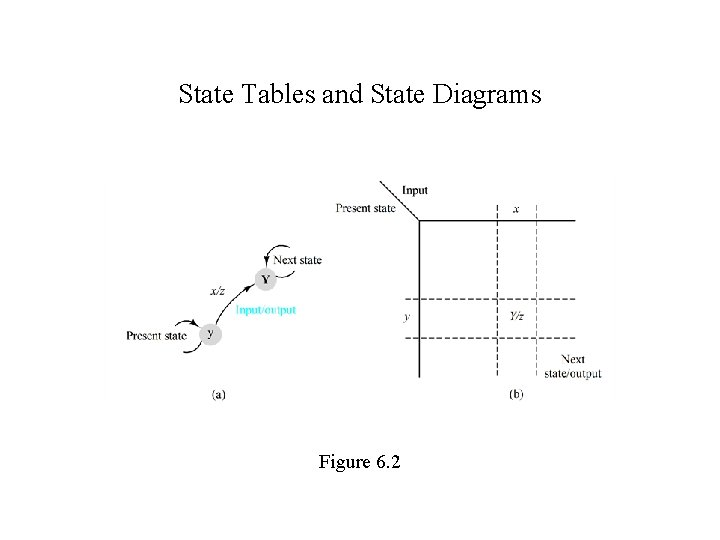State Tables and State Diagrams Figure 6. 2Sequential Circuit Example Figure 6. 3Latch and Flip-flop Timing Figure 6. 4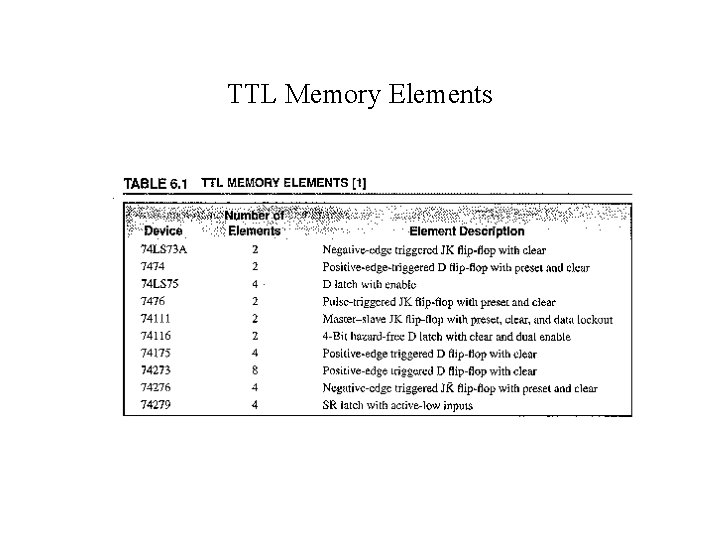TTL Memory Elements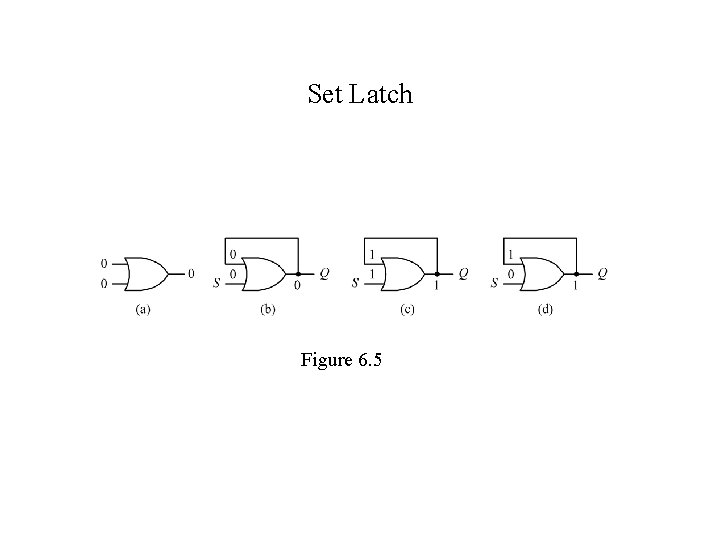Set Latch Figure 6. 5Reset Latch Figure 6. 6Set-Reset Latch (SR latch) Figure 6. 7NAND SR Latch Figure 6. 8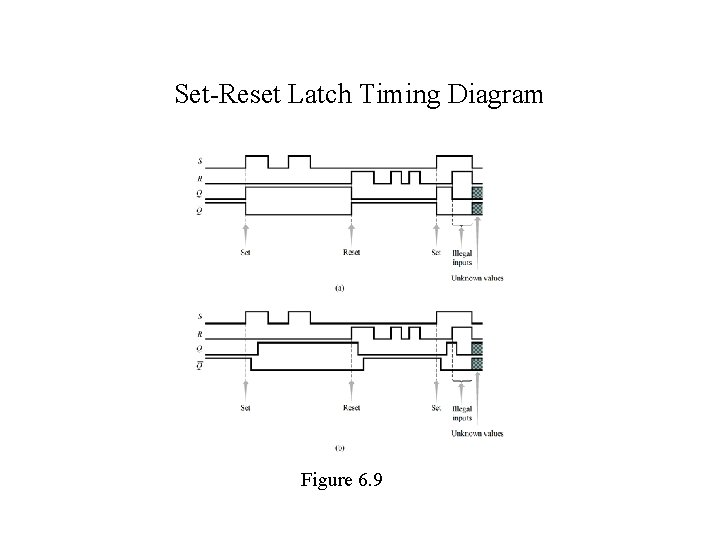Set-Reset Latch Timing Diagram Figure 6. 9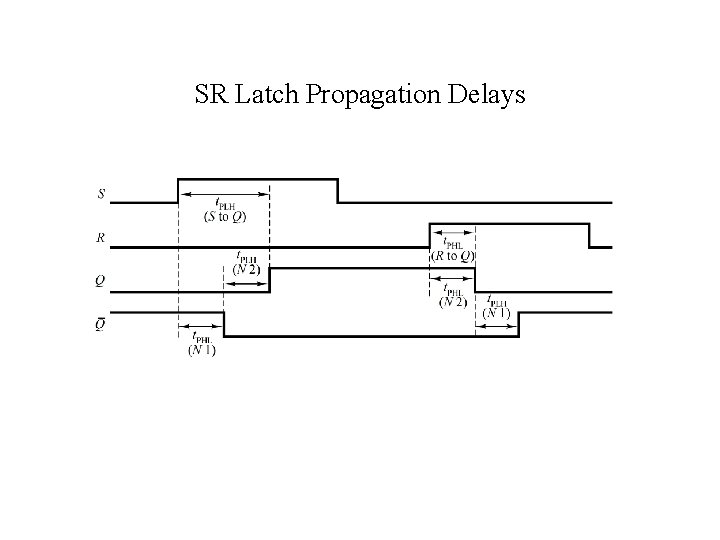SR Latch Propagation Delays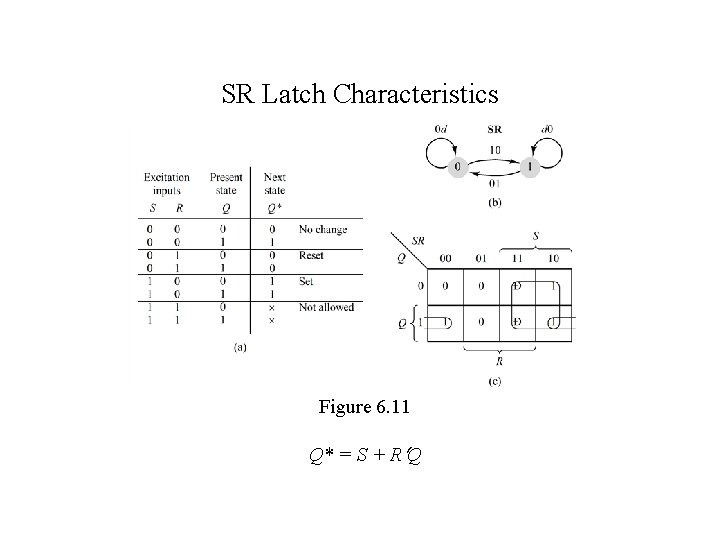SR Latch Characteristics Figure 6. 11 Q* = S + R QSN 74279 Latch with Two Set Inputs Figure 6. 12Gated SR Latch Figure 6. 13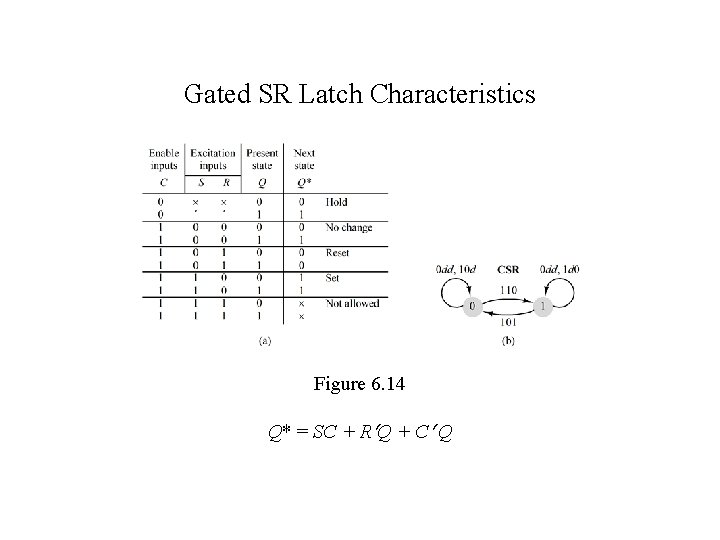Gated SR Latch Characteristics Figure 6. 14 Q* = SC + R Q + C Q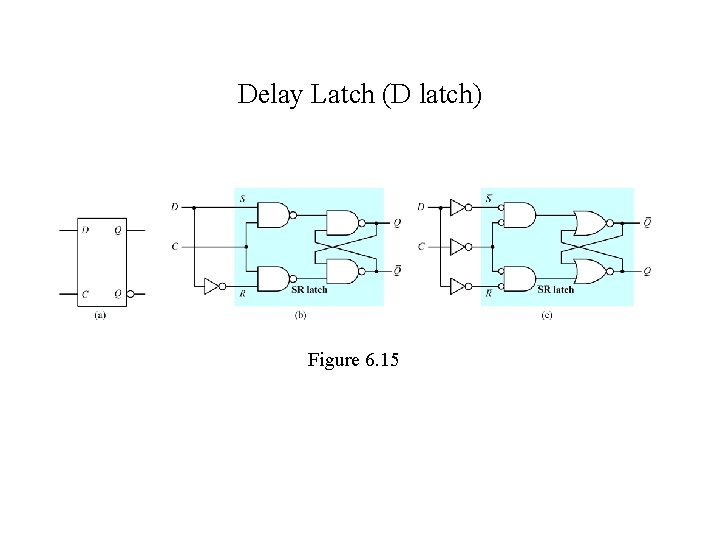Delay Latch (D latch) Figure 6. 15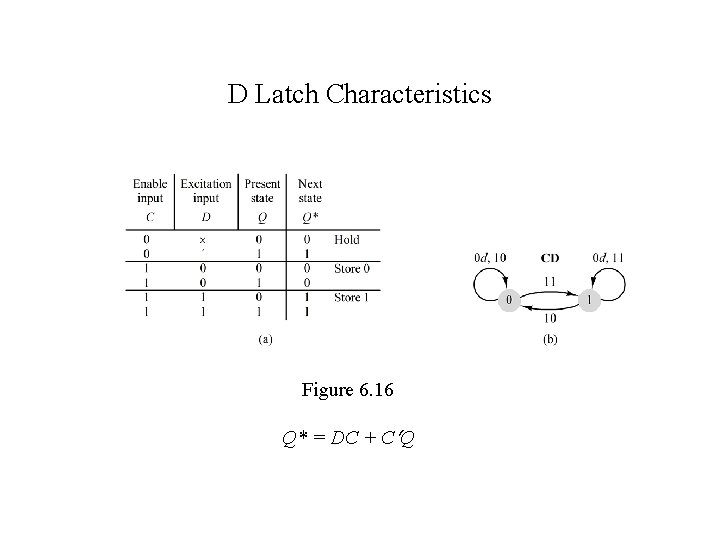D Latch Characteristics Figure 6. 16 Q* = DC + C Q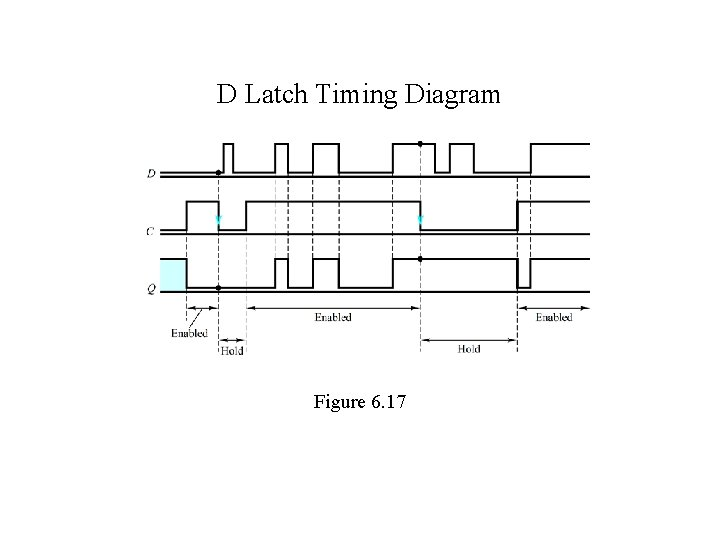D Latch Timing Diagram Figure 6. 17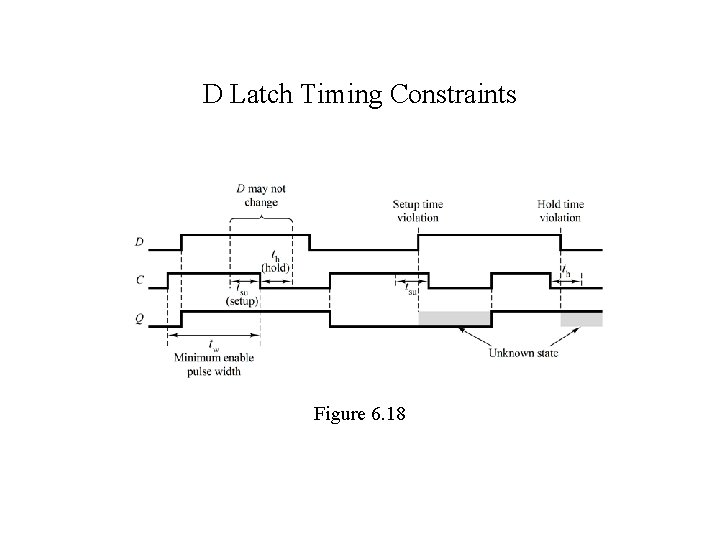D Latch Timing Constraints Figure 6. 18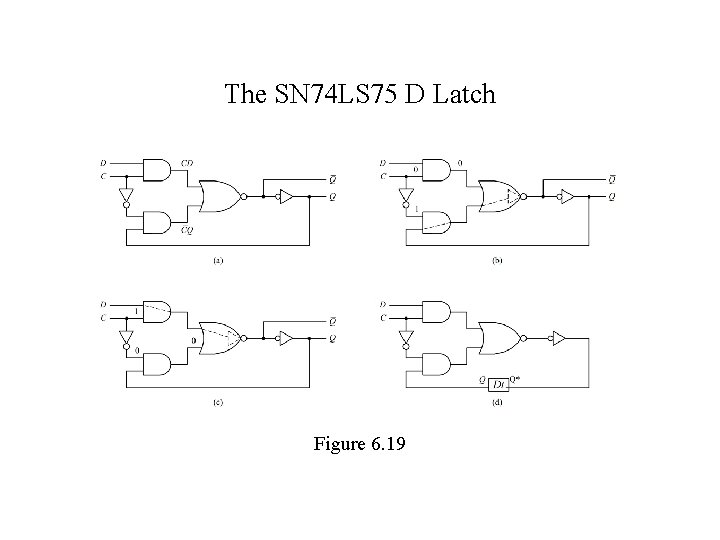The SN 74 LS 75 D Latch Figure 6. 19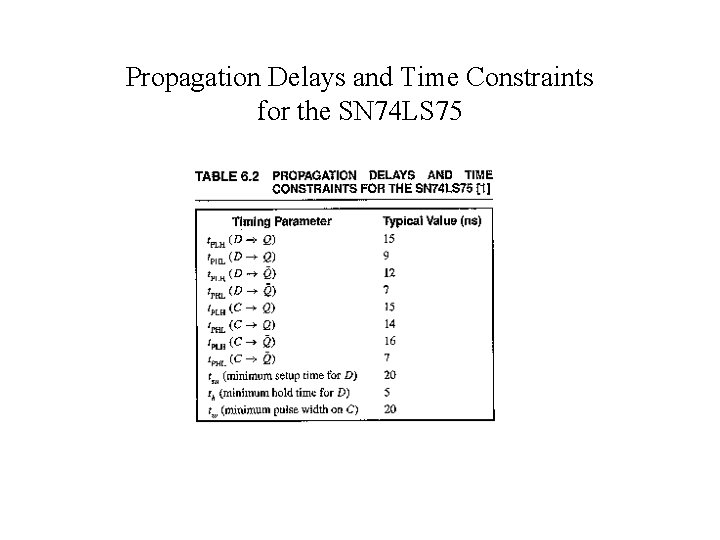Propagation Delays and Time Constraints for the SN 74 LS 75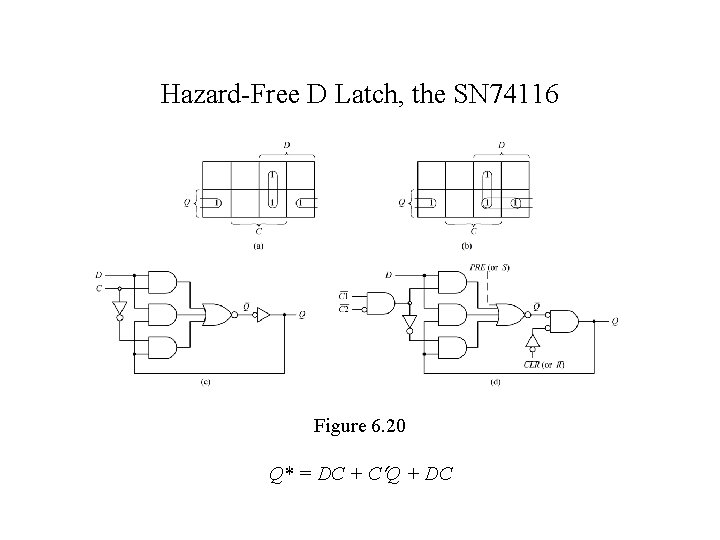Hazard-Free D Latch, the SN 74116 Figure 6. 20 Q* = DC + C Q + DC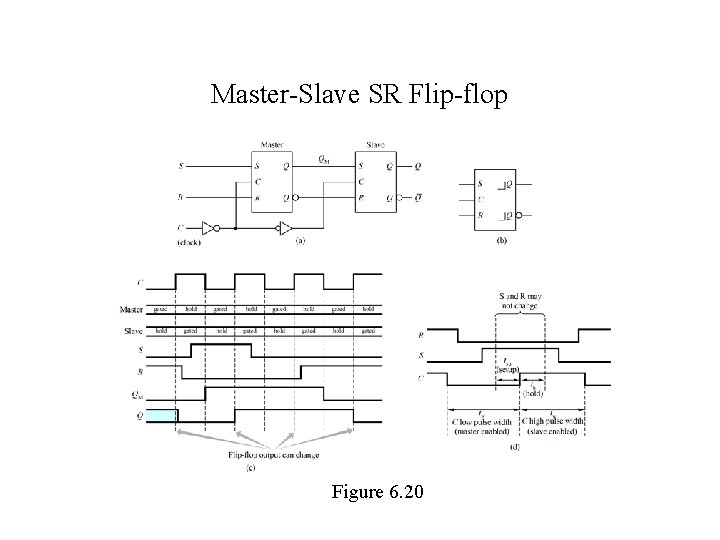Master-Slave SR Flip-flop Figure 6. 20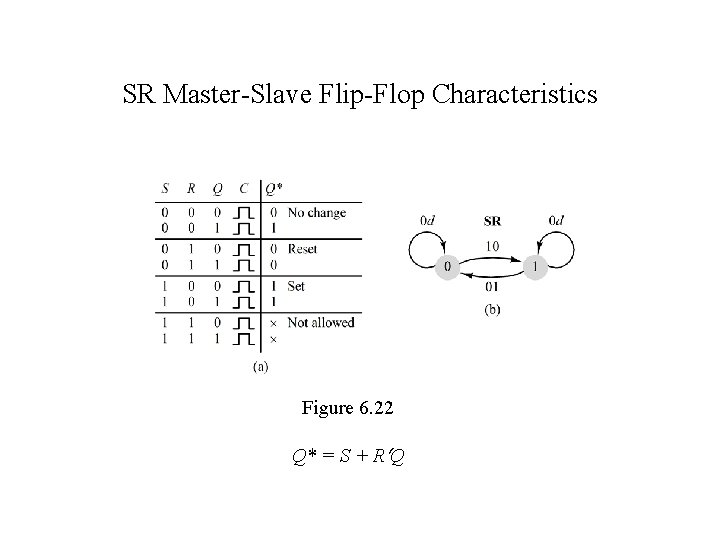SR Master-Slave Flip-Flop Characteristics Figure 6. 22 Q* = S + R QMaster-Slave D Flip-Flop Figure 6. 23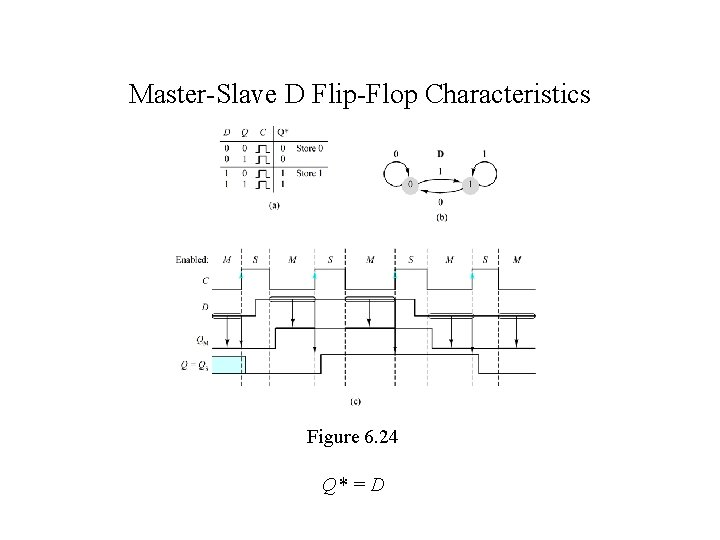Master-Slave D Flip-Flop Characteristics Figure 6. 24 Q* = D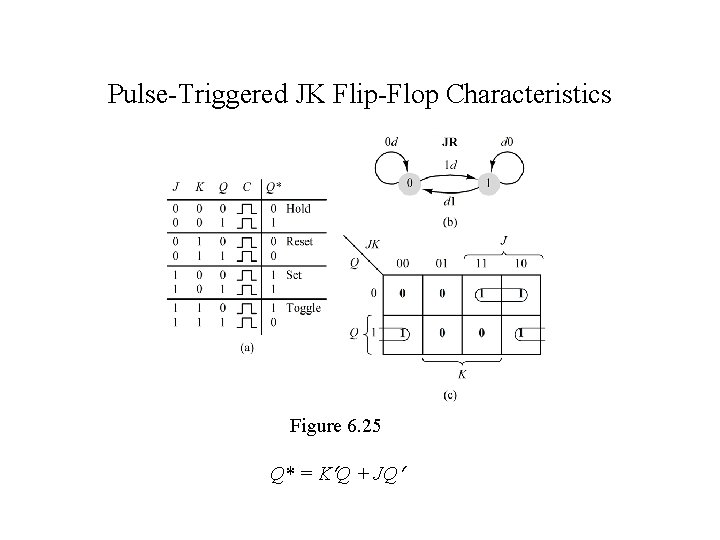Pulse-Triggered JK Flip-Flop Characteristics Figure 6. 25 Q* = K Q + JQPulse-Triggered JK Flip Realization Figure 6. 26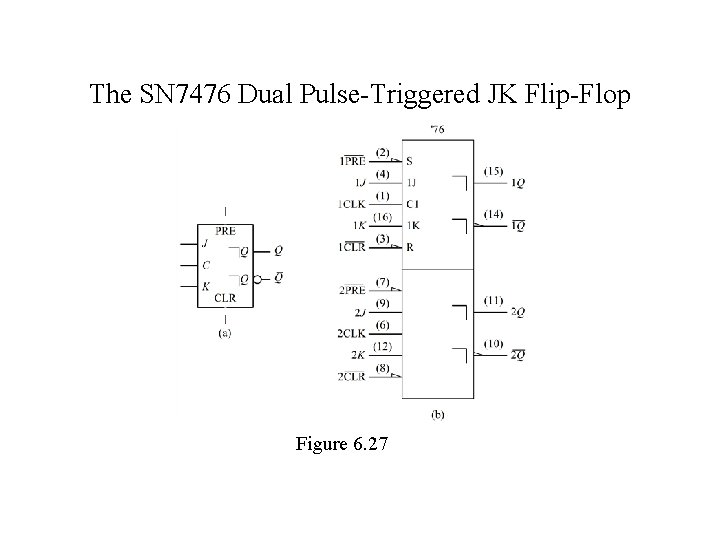The SN 7476 Dual Pulse-Triggered JK Flip-Flop Figure 6. 27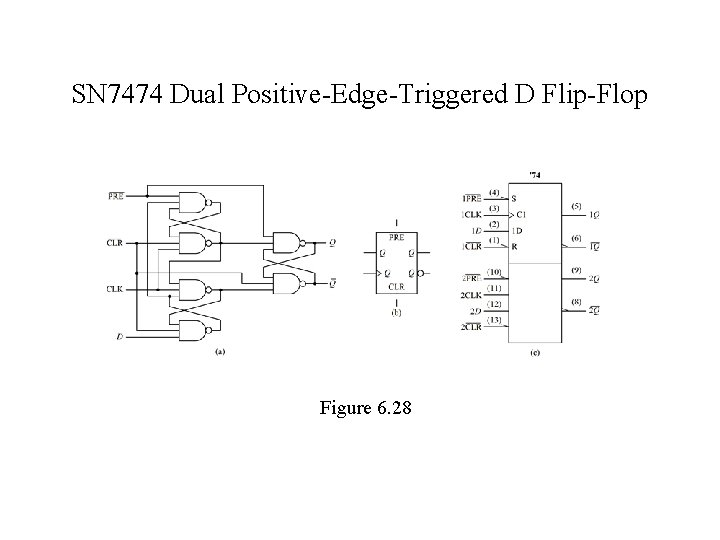SN 7474 Dual Positive-Edge-Triggered D Flip-Flop Figure 6. 28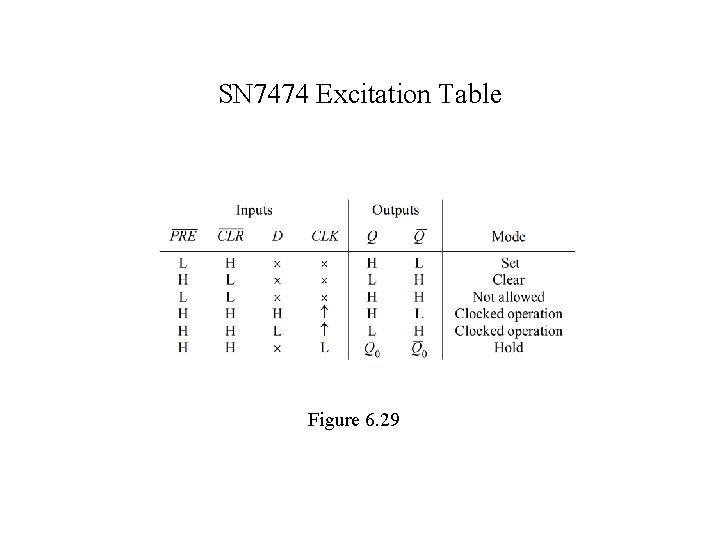SN 7474 Excitation Table Figure 6. 29SN 7474 Flip-Flop Timing Specifications Figure 6. 30SN 74175 Positive-Edge-Triggered D Flip-Flop Figure 6. 31 (a)SN 74273 Positive-Edge-Triggered D Flip-Flop Figure 6. 31 (b)SN 74 LS 73 A Edge-Triggered JK Flip-Flop Logic Diagram Figure 6. 32 (a)SN 74 LS 73 A Logic Symbols Figure 6. 32 (b) and (c)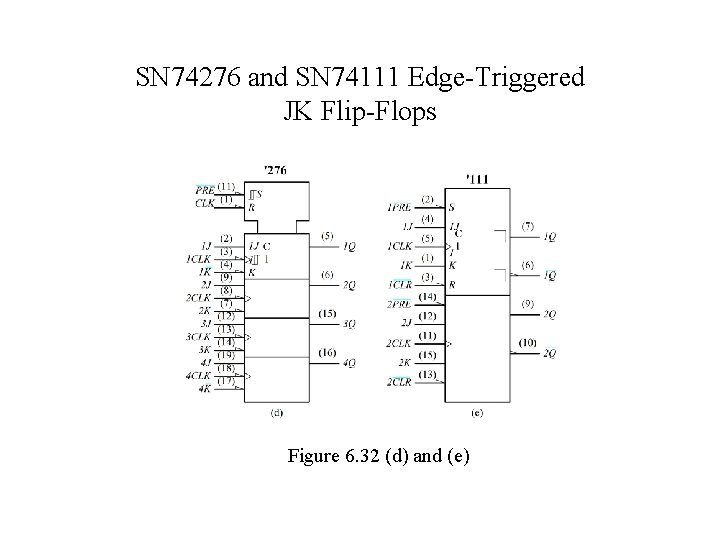SN 74276 and SN 74111 Edge-Triggered JK Flip-Flops Figure 6. 32 (d) and (e)Negative-Edge-Triggered T Flip-Flop Figure 6. 33Edge-Triggered T Flip-Flop Characteristics Figure 6. 34 Q* = QClocked T Flip-Flop Figure 6. 35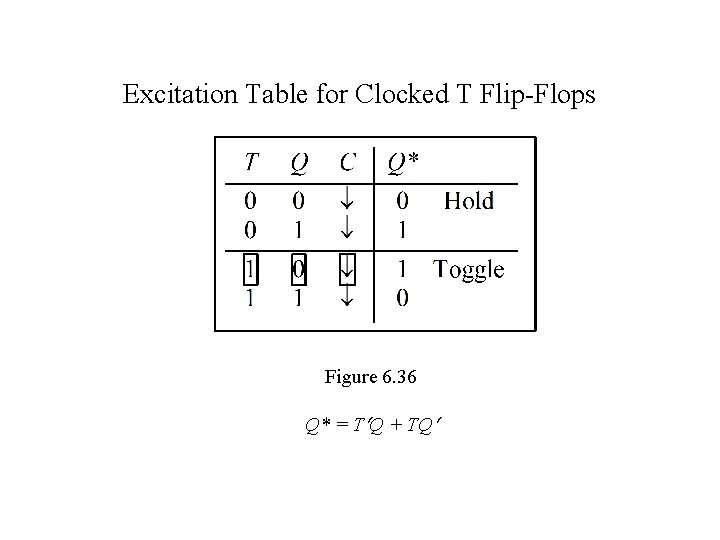Excitation Table for Clocked T Flip-Flops Figure 6. 36 Q* = T Q + TQThe Clocked T Flip-Flop Timing Diagram Figure 6. 37Summary of Latch and Flip-Flop Characteristics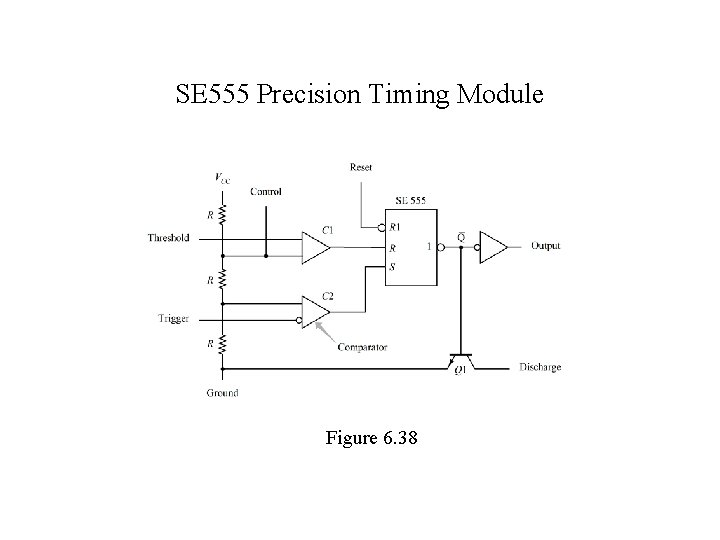SE 555 Precision Timing Module Figure 6. 38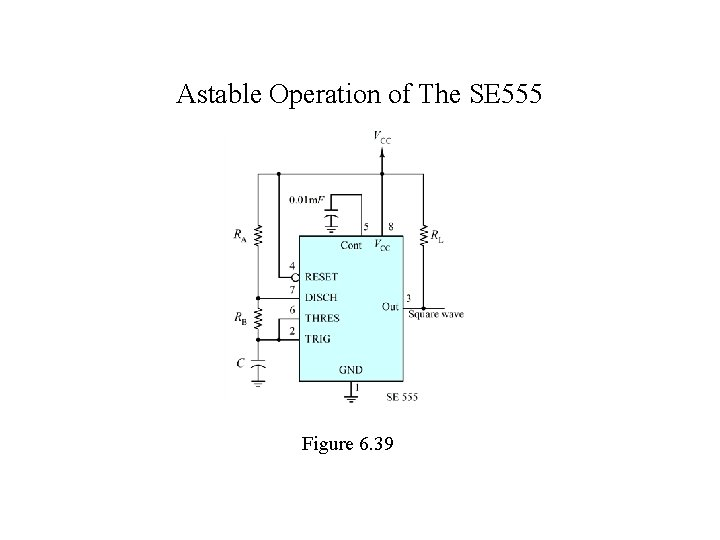Astable Operation of The SE 555 Figure 6. 39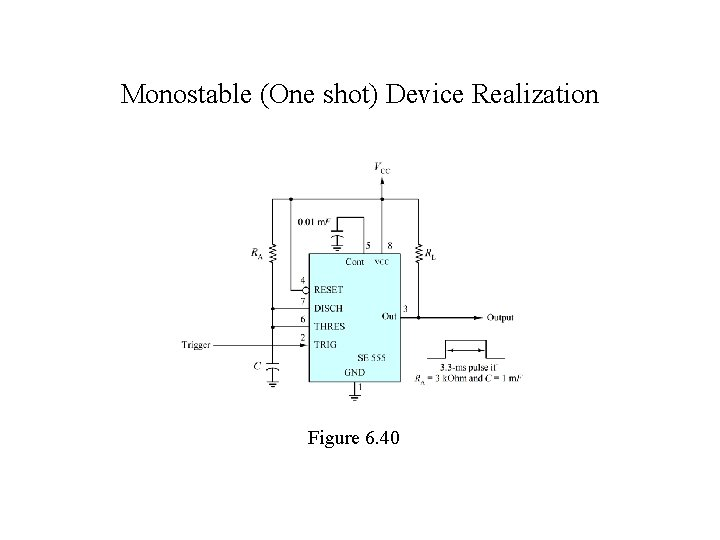Monostable (One shot) Device Realization Figure 6. 40PROM-based Sequential Circuits Figure 6. 41PROM-based Sequential Circuit Example Figure 6. 41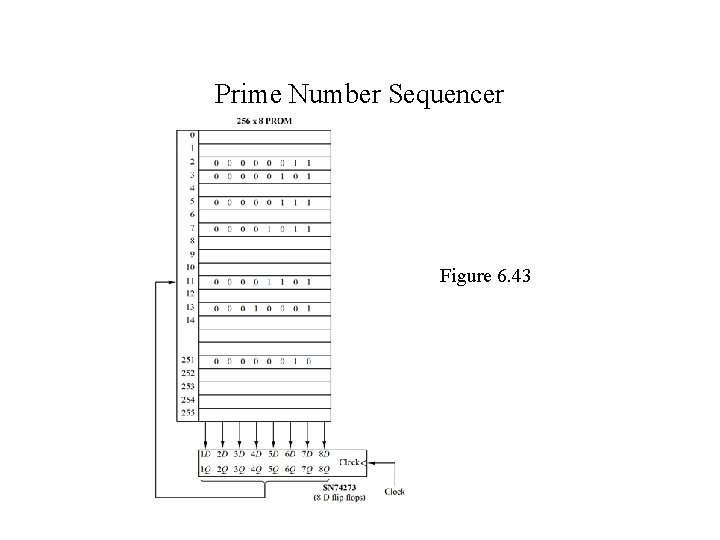Prime Number Sequencer Figure 6. 43